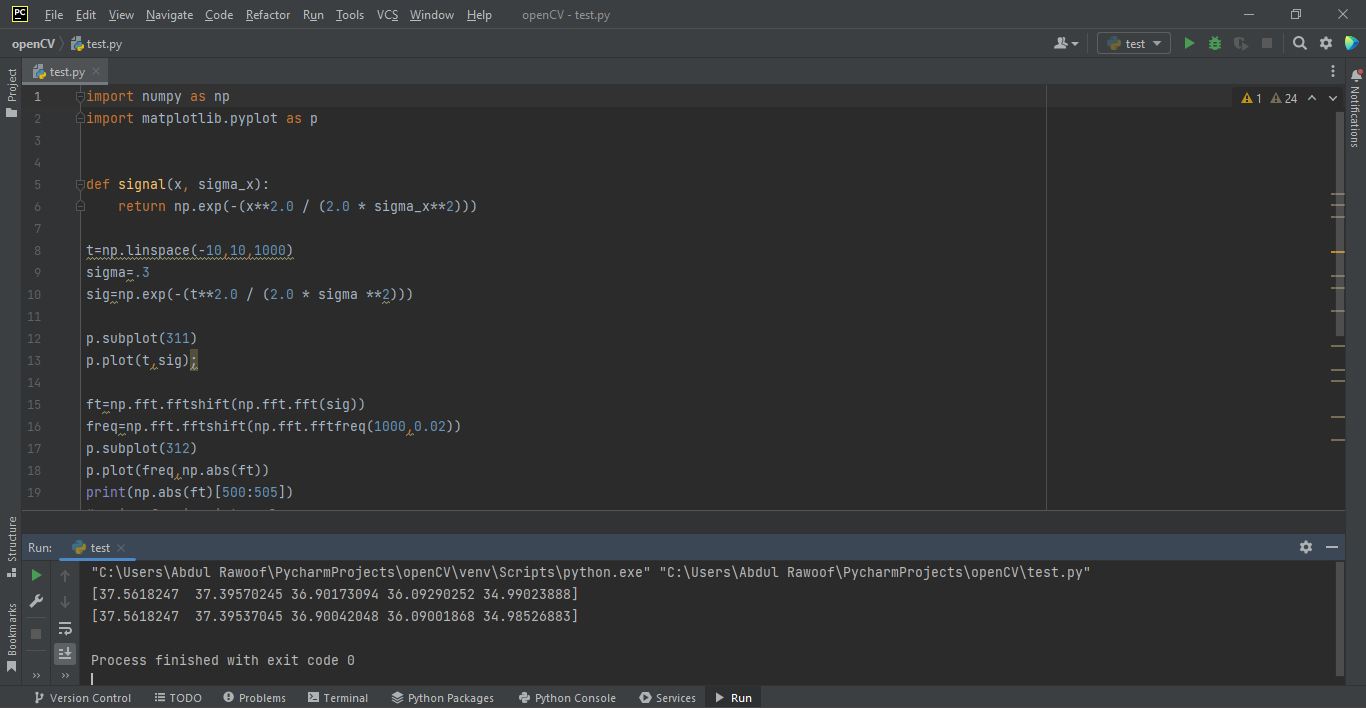# Computing the discrete Fourier transform (DFT) at the frequencies of FFTby Abdul Rawoof A RUpdated: Mar 27, 2023Solution Kit

The discrete Fourier transform (DFT) converts a finite sequence of equally spaced samples into a same-length sequence of equally spaced samples of the discrete-time Fourier transform (DTFT) function, a complex-valued function of frequency in Python.

In Python, the fast Fourier transform (FFT) is an algorithm for computing the discrete Fourier transform (DFT), whereas the discrete Fourier transform is the transform itself. Another distinction that we'll see made in the scipy.fft library is between various types of input. fft() method that accepts complex-valued input, and rfft() method that accepts real-valued input. It can calculate a signal's frequency spectrum and directly examine information or data encoded in the component sinusoids' phase, frequency, and amplitude. For example, human speech and hearing use signal with this encoding type. It differs from the discrete-time Fourier transform (DTFT) in that its input and result sequences are both finite and therefore said to be the Fourier analysis of finite-domain or periodic discrete-time functions.

Here is an example of how to compute Discrete Fourier Transform(DFT) at the frequencies of FFT in Python:Fig: Preview of the output that you will get on running this code from your IDE.

### Code

In this solution we're using NumPy and Matplotlib libraries.

### Instructions

Follow the steps carefully to get the output easily.

1. Install PyCharm Community Edition on your computer.
2. Open terminal and install the required libraries with following commands.
3. Install NumPy - pip install numpy.
4. Install Matplotlib - pip install matplotlib.
5. Create a new Python file(eg: test.py).
6. Copy the snippet using the 'copy' button and paste it into that file.
7. Run the file using run button.

Note: Remove the third line of the code(%matplotlib inline).

I hope you found this useful. I have added the link to dependent libraries, version information in the following sections.

I found this code snippet by searching for 'computing dft at frequencies of fft' in kandi. You can try any such use case!

### Environment Tested

I tested this solution in the following versions. Be mindful of changes when working with other versions.

1. The solution is created in PyCharm 2022.3.3.
2. The solution is tested on Python 3.9.7.
3. NumPy version 1.24.2.
4. Matplotlib version 3.7.1.

Using this solution, we are able to compute discrete fourier transform at the frequencies of fft in Python with simple steps. This process also facilities an easy way to use, hassle-free method to create a hands-on working version of code which would help us to compute discrete fourier transform at the frequencies of fft in Python.

### Dependent Libraries

numpyby numpy

Python23755Version:v1.25.0rc1The fundamental package for scientific computing with Python.

Support
Quality
Security
Reuse

numpyby numpy

Python23755Version:v1.25.0rc1License: Permissive (BSD-3-Clause)

The fundamental package for scientific computing with Python.
Support
Quality
Security
Reuse

matplotlibby matplotlib

Python17559Version:v3.7.1License: No License (null)

matplotlib: plotting with Python

Support
Quality
Security
Reuse

matplotlibby matplotlib

Python17559Version:v3.7.1License: No License

matplotlib: plotting with Python
Support
Quality
Security